# 1/t (Once upon a time)

I mentioned last month my new “unit circle” clock, which marks the hours in terms of their radian angle measure and parametrizes time positively, which is to say, counterclockwise.   (For example, the clock below reads 4:05:40.)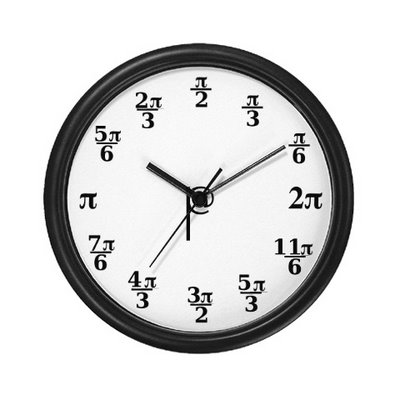One of the unexpected pleasures of such a clock is the usual effect it has on students, namely, panic.   Many of them simply do not realize it is not a standard clock, and find the utterly inexplicable location of its hands a source of temporal discomfort (or, as one student more succinctly put it, “Holy shit!   I can’t tell if I’m really late for my next class… or really early for it!”).   Others eventually realize how the clock functions, but find it only taunts them and their tenuous grasp of trigonometry.

So of course I’m now on the look out for a new, similarly awesome and befuddling math clock.   Here are ten of the better ones I’ve found whilst scouring the internets.

Version 1: The square clock. No, it’s not a clock in the shape of a rhombic parallelogram (although we will end with one of those).   Instead, it’s a standard clock whose twelve numbers have been replaced by equivalent root-of-a-perfect-square quantity.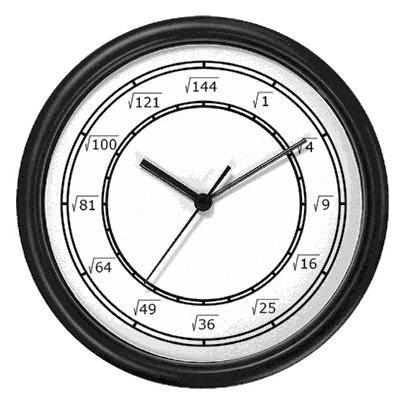It’s not particularly mathy, but it does have an understated elegance about its design, and I do think it has a punny name.

Version 2: The algebra clock. Whereas my clock is all about basic trigonometry, here’s a clock that’s all about algebra, with each of the twelve numerals of the clock replaced with an arithmetic or algebraic expression representing it.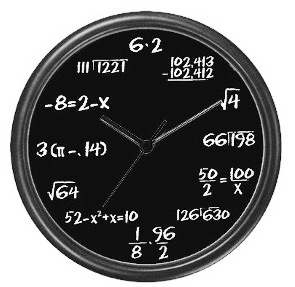Unfortunately, I’m not particularly fond of this clock for two main reasons.

First off, this clock is internally inconsistent: most of the expressions are simply arithmetic expressions that quite literally are the numbers they’ve replaced (for example, the expression$displaystyle frac{1}{8} cdot frac{96}{2}$ is, in fact, exactly 6), while the positions for 4, 7,   and 10 o’clock are instead algebraic expressions which yield the correct value as a solution.   A better, or at the very least, more consistent design might have been to replace, say, the 10 o’clock position with “x if   -8 = 2 – x,” and so forth.

But even still the clock would not be well-designed, which my second gripe.   For example, the arithmetic expression “3($pi$ – 0.14)” is only approximately 9; too many students already think that$pi$ is exactly 3.14 already!   Also, the polynomial at 7 o’clock has two solutions, namely, x = 7 and x = -6, and the latter (on a clock, at least) is equivalent to 6.

Phooey on this clock, and its many variants (such as the desktop model below).Version 3: The three nines clock.   A chronographical variation of the popular Four Four Problem, each number on this clock is replaced by an equivalent arithmetic expression involving exactly three 9s.I even like the fact that the symbol in the middle can be interpreted as a symmetric depiction of three nines, each with a common “center” and rotated 120 degrees from its nearest neighbor.   Sweet clock.

Version 4. The number system clock. Here’s a clock in which each of the numbers have been replaced by equivalent arithmetic or notational expressions, which gives one a nice mathematical workout.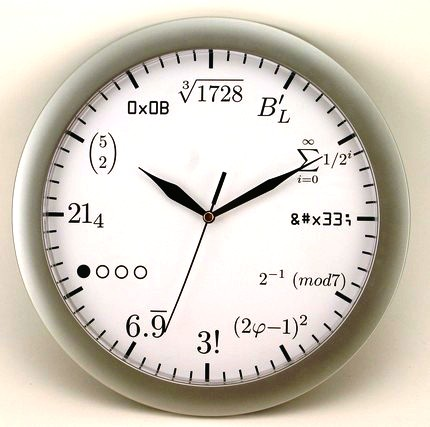For example, some of the more interesting ones include

• 1 represented as Legendre’s constant
• 3 represented in XML unicode (for web geeks)
• 4 represented as an element in the finite field of order 7
• 5 represented as an arithmetic consequence of the Golden Ratio
• 8 represented in symbolic binary
• 9 represented in Base 4
• 10 represented as a binary coefficient

Version 5. A mathematical constant clock. This is a clever clock which no longer attempts to replace the values 1, 2, 3, … 12 with equivalent expressions, but instead includes several important (and not so important)   mathematical constants close to these values along the periphery of the clock in their correct positions, tacitly assuming the circumference of the clock measures exactly 12 units.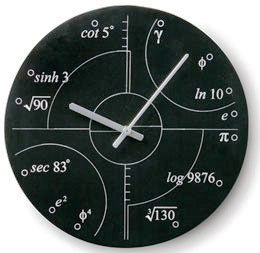We find along the way

• The Euler-Mascheroni constant$gamma$, which is approximately 0.6.
• The Golden Ratio$phi$, which is approximately 1.6, as well as later its fourth power, which is approximately 6.9.
• The natural logarithm of the decimal base ln(10), which is approximately 2.3.
• Euler’s constant e, which is approximately 2.7, as well as later its square, which is approximately 7.4
• Archimedes’ constant$pi$, which is approximately 3.1.
• The common logarithm of 9876, which is approximately 4.0.
• The cube root of 130, which is approximately 5.1.
• The secant of 83 degrees, which is approximately 8.2.
• The square root of 90, which is approximately 9.5.
• The hyperbolic sine of 3, which is approximately 10.1.
• The cotangent of 5 degrees, which is approximately 11.4.

I dig the combination of precision (of placement) and imprecision (of the hour markers)   in this design.

Version 6. The physical constant clock. A variation on the previous theme, it replaces the values 1, 2, 3, … 12 with corresponding important (and not so important)   scientific constants close to these values, with bullets along the periphery in their exact locations relative to the assumed 12-unit length of the circumference.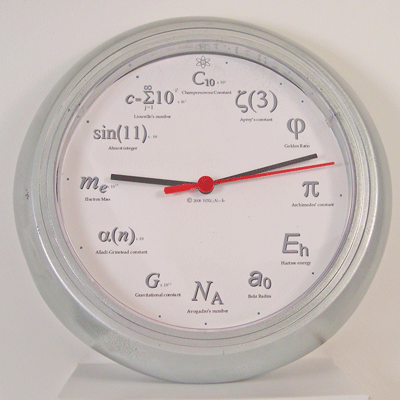Dusting off my physics book, I find the values are

• The Champernowne constant C10, which is about 12.3 (times 10-2).
• Apery’s constant$zeta(3)$, which is about about 1.2.
• The Golden Ratio$Phi$ — misrepresented as$varphi$ on the clock — which is approximately 1.6.
• Archimedes’ constant$pi$, which is approximately 3.1.
• A Hartree Eh, which is about 4.4 (times 10-18 joules).
• The Bohr radius a0, which is about 5.3 (times 10-12 meters).
• The gravitational constant G, which is about 6.7 (times 10-11 cubic meters per kilogram per square second).
• The Alladi-Grinstead constant$alpha(n)$ (or rather, it’s limit as n tends to infinity), which is about 8.1 (times 10-1).
• The electron mass me, which is about 9.1 (times 10-31 kilograms).
• The sin of 11 radians, which apparently is famous for being almost exactly -1.
• Liouville’s constant c, which is about 11 (times 10-2).

Damn, that was a mental workout.

Version 7. Binary clock.   This is a nifty LED clock that displays the time in two forms of binary.   If one chooses a vertical display mode, then time is displayed in its usual base 10 form, with the individual digits represented in their binary form.   For example, under this interpretation, the clock below reads 12:01:47.However, the clock also has a horizontal display mode, in which each of the rows display the hours, minutes, and seconds in pure binary, which is significantly cooler.   For example, under this interpretation, the clock above reads 03:17:37.   That’s as cool (in terms of awesomeness and befuddlitude) as my trig clock.

Version 8. Recursive clock. Not really mathematical, but hilariously tautological.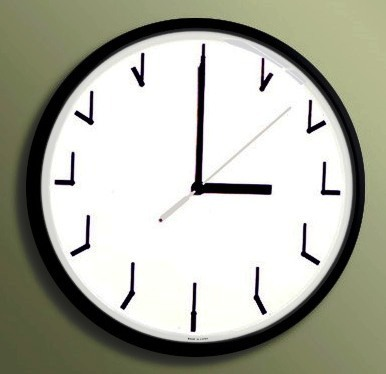Version 9. Non-cyclic clock. To hell with all that base-12 modular arithmetic we need to do on a daily basic!   Why not just have a clock that includes every individual minute from now until eternity: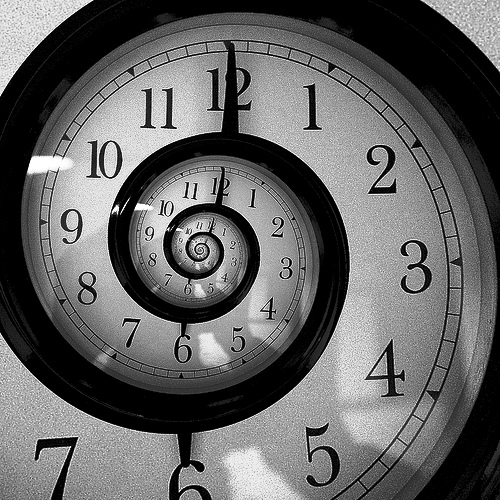Unfortunately, I suspect this clock would be a bitch to hang.

Finally, Version 10, for when you just don’t care for time-keeping conventions anymore.See you next time… however you keep time.

<a href=”Notes-10.pdf”>
This entry was posted in nerdify. Bookmark the permalink.

### 4 Responses to 1/t (Once upon a time)

1.J. Norman Wells says:

Sirs,
I am trying to locate a place where I can purchase
the “Version 5. A mathematical constant clock” shown
above. Can you help me.
J. Norman Wells

2.John Foggitt says:

Thanks for assembling such a wonderful collection of curious clocks.

One that’s missing (my idea for a clock – I’ve not seen or heard about such a clock) is a Complex Number Clock. The numbers from One to Twelve would read:
1 0.5 + 0.866 i
2 0.866 + 0.5 i
3 1
4 0.866 – 0.5 i
5 0.5 – 0.866 i
6 – i
7 – 0.5 – 0.866 i
8 – 0.866 – 0.5 i
9 – 1
10 – 0.866 + 0.5 i
11 – 0.5 – 0.866 i
12 i

It would be preferable to use the mathematical symbols to represent the numbers shown above in decimal form as ‘one half’ for 0.5 and ‘root 3 divided by 2’ for 0.866.

Are you aware of any supplier of customised clock faces whereby the purchaser can supply an image to be printed onto a clock face? If so I’d like to know the details.

3.John Foggitt says:

Has anyone ever considered decimalising time? We could have 100 seconds to the minute, 100 minutes to the hour and 10 hours to the day resulting in 100,000 seconds to the day instead of the current 86,400. This would mean a second would be 0.864 of its current value. Then everything depending on time would have to be recalculated. e.g. frequencies of radio and sound, speeds, in particular the speed of light, etc. This would be such an enormous task that I can’t see it coming about although we could use decimal time for everyday use and keep “old seconds” for scientific purposes, or maybe invent new terminology so that “second” would retain its current meaning. How about:
1 new second = 10 microdays,
1 new minute = 1 milliday
1 new hour = 1 deciday

•John Aidun says:

Dear John,

Early in the French revolution, when the metric system was being laid out and adopted, the French established decimal time. You can read about it in the book, “The Measure of All Things” by Ken Alder.

cheers, jba